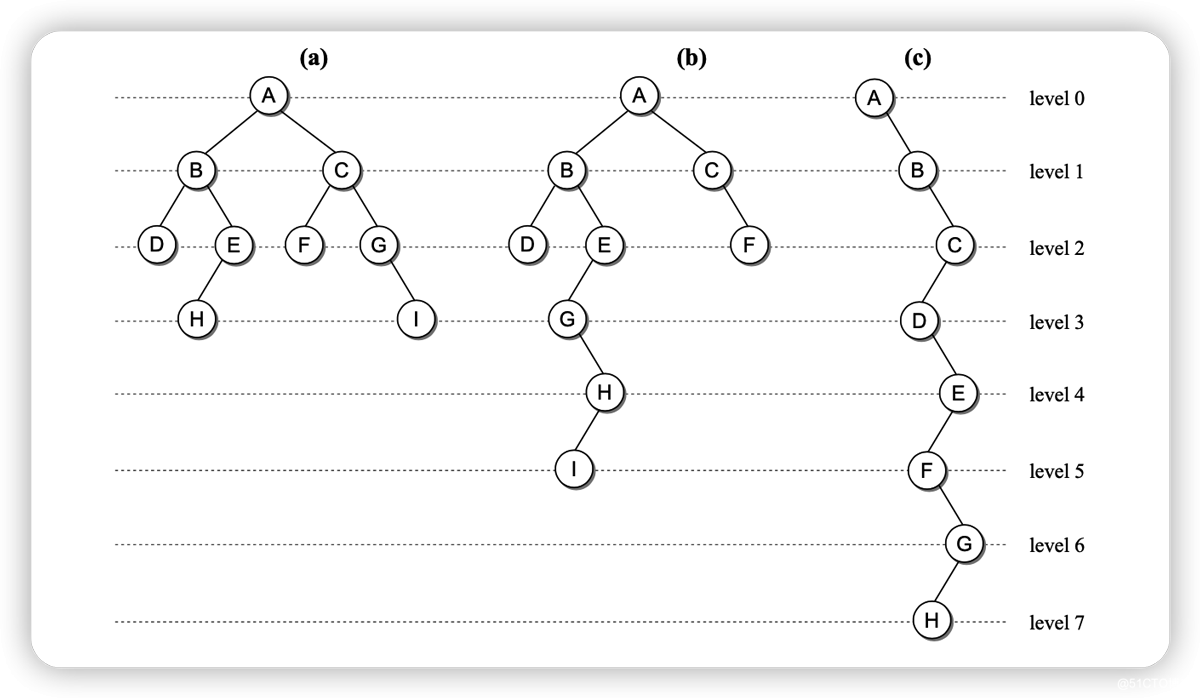﻿ Go 数据结构之二叉树详情_Golang_脚本之家
Golang# Go 数据结构之二叉树详情## Go 语言实现二叉树

### 定义二叉树的结构

```package main
import (
"fmt"
"math/rand"
"time"
)
type Tree struct {
Left *Tree
Value int
Right *Tree
}```

### 二叉树遍历

```func traverse(t *Tree) {
if t == nil {
return
}
traverse(t.Left)
fmt.Print(t.Value, " ")
traverse(t.Right)
}```

`traverse()`函数通过递归方式访问二叉树的全部节点。

### 创建二叉树

```func create(n int) *Tree {
var t *Tree
rand.Seed(time.Now().Unix())
for i := 0; i < 2 * n; i++ {
temp := rand.Intn(n * 2)
t = insert(t, temp)
}
return t
}```

### 插入值

`insert` 函数：

• 第一个 if 语句在插入时如果是空树，就把插入的节点设置为根节点，并创建为 `&Tree{nil, v, nil}`
• 第二个 if 语句确定插入值是否已在二叉树中存在。若存在，函数将直接返回且不执行任何操作
• 第三个 if 语句检查插入的值位于当前节点的左孩子节点还是右孩子节点，并插入到相应的位置。
```func insert(t *Tree, v int) *Tree {
if t == nil {
return &Tree{nil, v, nil}
}
if v == t.Value {
return t
}
if v < t.Value {
t.Left = insert(t.Left, v)
return t
}
t.Right = insert(t.Right, v)
return t
}```

## 测试

```package main
import (
"fmt"
"math/rand"
"time"
)
type Tree struct {
Left *Tree
Value int
Right *Tree
}
func traverse(t *Tree) {
if t == nil {
return
}
traverse(t.Left)
fmt.Print(t.Value, " ")
traverse(t.Right)
}
func create(n int) *Tree {
var t *Tree
rand.Seed(time.Now().Unix())
for i := 0; i < 2*n; i++ {
temp := rand.Intn(n * 2)
t = insert(t, temp)
}
return t
}
func insert(t *Tree, v int) *Tree {
if t == nil {
return &Tree{nil, v, nil}
}
if v == t.Value {
return t
}
if v < t.Value {
t.Left = insert(t.Left, v)
return t
}
t.Right = insert(t.Right, v)
return t
}
func main() {
tree := create(10)
fmt.Println("The value of the root of the tree is", tree.Value)
traverse(tree)
fmt.Println()
tree = insert(tree, -10)
tree = insert(tree, -2)
traverse(tree)
fmt.Println()
fmt.Println("The value of the boot of the the tree is", tree.Value)
}```

The value of the root of the tree is 18
0 1 4 5 6 8 9 10 12 14 15 18 19
-10 -2 0 1 4 5 6 8 9 10 12 14 15 18 19
The value of the boot of the the tree is 18